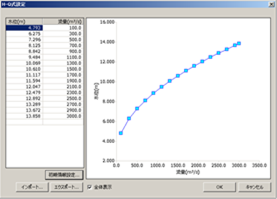# FAQ/ Give multiple HQ formulas to a river channel cross-section¶

Can multiple HQ expressions be set for a single river channel cross-section?

## response¶

It's possible.

You can set the HQ formula for each section.

The HQ expression is the expression

$Q = a (H + b)^2$

You can specify a factor of $$a$$ and a factor of $$b$$ in .
In addition, the range of flow rates to which the HQ formula is applied (upper flow rate applied by HQ formula, lower flow rate applied by HQ formula) can be set.
In addition, you can choose to apply the HQ formula only to the positive flow rate or to apply the HQ formula to the negative flow rate as well.

Alternatively, you can apply a tabular HQ expression (HQ conversion table) instead of an HQ expression.Figure: Tabular HQ expression setting screen

For details, please refer to Technical Reference Function to Correct Water Level with HQ Formula and FAQ/What is HQ Formula?

Last update: 2023-03-17